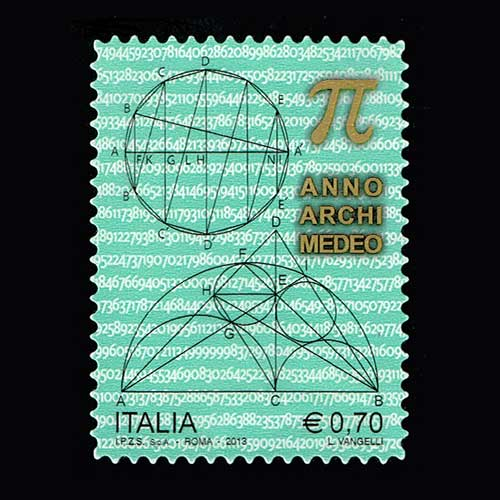Celebrating world Pi day

14 Mar 2018  Wed

Pi is one of the most interesting constants of mathematics. It has been the subject of fascination for many mathematicians from ancient times. Pi is generally associated with a circle, but it’s also used in geometry, trigonometry, analysis, etc. Today world is celebrating Pi day because of the month and day in the calendar is 3/14 and the first three digits of the mathematical formula of Pi is also 3.14.

Pi was known in the Indian subcontinent since 3000 BC. Indian mathematicians were the first to obverse the circumference of a circle increases in proportion to its diameter. Hence, the ancient Indians established a relation called “perimeter/diameter= constant”, this constant was Pi but India didn’t call it so. The value of Pi was documented from the time Rig-Veda was written. The value of pi went through many evolutions and changes to reach an approx value. In 476 AD, the greatest Indian mathematic Aryabhatta found the approx value of Pi as 3.1416 in four decimal places.

The Babylonians found the value of Pi in three decimal places around 1900 to 1680 BC. Later on, ancient Egypt mathematically calculated the formula of the area of a circle to an approx value of 3.1605. After this came a mathematician from Syracuse called Archimedes in 287 BC. He is known as the great mathematician of the ancient world, it was his calculation that gave the world the approx value of pi between 3 1/7 and 3 10/71 fractions.

A similar approach like Archimedes was used by the Chinese mathematician called Zu Chongzhi his calculation of Pi is in the ratio, it is a brilliant value to use in computers today. In the 1700s the Pi was represented in Greek letter. William Jones used this letter in 1706, but it was popularized by Leonhard Euler in 1737.

The fascination behind Pi has lasted longer than any civilization, the power of human curiosity and knowledge as made it a symbol of intelligent in history.

Italy Post has also issued a postage stamp in 2013 to celebrate the 2300th anniversary of the birth of Archimedes, who calculated the approx value of Pi. To Know more about the Indian mathematician Aryabhatta click here.

Image Courtesy: Ebay.com